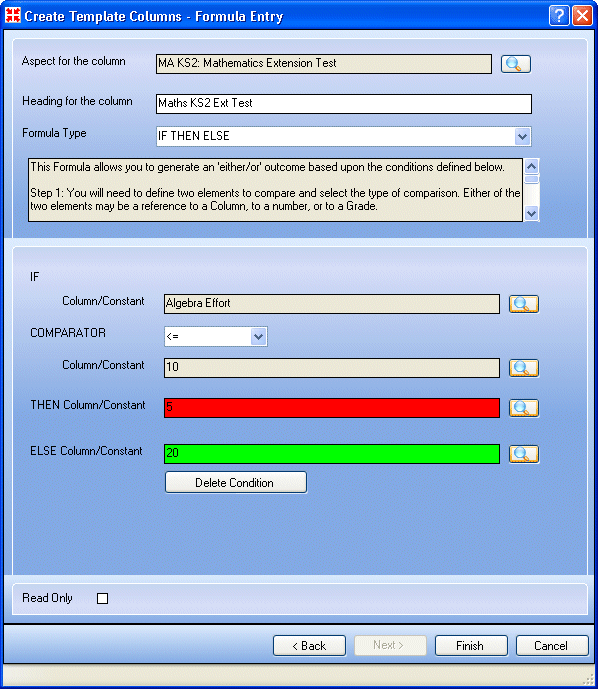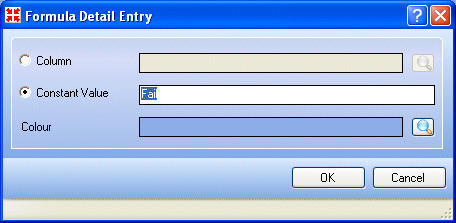# Inserting a Grade Tally Columns Formula

 Page 71/209 Date 08.07.2018 Size 12.14 Mb.

### Inserting a Grade Tally Columns Formula

This formula is only available for use where the outcome of the calculation is stored in a Marks (Integer), Marks (Decimal) or Comments aspect.

This formula will search across the selected columns and return the number of results that can be found in the database regardless of time frame. You need to identify the grade or grades in which you are interested (multiple grades should be separated by a comma, e.g. A, B, C). If the search finds 3 x A, 4 x B, 7 x C then the outcome is 14.

1. Select Grade Tally Columns from the Formula Type drop-down list.

2. Select columns to be tallied (please see Choosing a Selection of Columns on page 143).

3. Specify the grades to be counted, separating multiple grades with a comma.

NOTE: You must include every grade that you wish to be tallied. Entering A will only count the number of A’s. If you also wish to count A+ and A-, you must include these in your list.
1. ### Inserting a Grade Tally Historic Formula

This formula is only available for use where the outcome of the calculation is stored in a Marks (Integer), Marks (Decimal) or Comments aspect.

This formula will search the selected grade aspect column and return the number of the chosen grade that can be found within a specified date range. You must select a grade to be counted and specify a date range through which the grades will be counted. You then need to choose whether the count should be based on results greater than or equal to the selected grade.

1. Select Grade Tally Historic from the Formula Type drop-down list.

2. Choose the required column by clicking the Field Browser button to display the Template Columns dialog.

3. Click the required column name then click the Select button.

4. Enter the required dates in the From and To fields or click the appropriate drop-down arrow to select the required date from the calendar.

5. Choose the type of comparison by clicking the appropriate radio button.

6. Select the required grade form the drop-down list.

NOTE: The position of the grade is based on its location in the grade set, not alphabetically, i.e. if the grades are listed Z, A, B, C, etc. then Z is deemed to be higher than A.
1. ### Inserting a Marks to Grade Formula

This formula is only available for use where the outcome of the calculation is stored in a grade aspect.

This formula generates a grade based on the result found in the selected column. The grades that can be generated by this formula are limited to grades listed in the grade set attached to the selected column. The formula uses any values defined in the grade set or values that you supply when setting up the formula. Example:

A = 100
B = 75

C = 50

When calculating the formula, if a result of 75 is found, then a B is returned in the outcome cell. If a value of 50 is found, then a C is returned and so on.

NOTE: No rounding is applied to this formula and therefore, to attain a grade, the value must be at least that required. Using the previous example, a B can only be achieved if the value is greater than or equal to 75. Values between 50 and 74 will all return Cs.

1. Select Marks to Grade from the Formula Type drop-down list.

2. Choose the required column by clicking the Field Browser button to display the Template Columns dialog.

3. Click the required column name then click the Select button.

4. Any default values in the grade set found for the chosen aspect are displayed in the Mark column. These can be overtyped or you can enter your own values.

NOTE: No rounding is applied during this calculation. To attain a grade, the threshold value must be reached. When reference is made to an empty cell in a marksheet, a blank outcome is generated.
1. ### Inserting an If Then Else Formula

This formula is only available for use where the outcome of the calculation is stored in a grade, Marks (Integer), Marks (Decimal) or Comments aspect.

This formula enables you to generate an either/or outcome based upon the conditions you select. You need to specify the two values to be compared, then define the two possible outcomes depending on whether the comparison is true or false.

For example:

If aspect A is greater than grade C, then aspect B = Pass else aspect B = Fail.1. Select If Then Else from the Formula Type drop-down list.

2. Choose or enter the IF column/constant value (please see Selecting Column/Constant Values on page 142).

3. Select the Comparator from the drop-down list such as >, >=, =, etc.

4. Choose or enter the column/constant to compare against.

5. Choose or enter the THEN column/constant, e.g. Pass. Colour coding can also be applied by clicking the Field Browser button by the Colour field.

6. Choose or enter the ELSE column/constant, e.g. Fail. Colour coding can also be applied by clicking the Field Browser button by the Colour field. This field is mandatory – you must enter a value.NOTE: When reference is made to an empty cell in a marksheet, a blank outcome is generated.